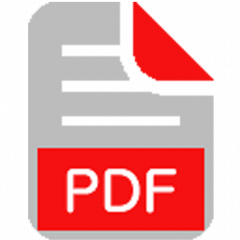UART INTERFACING WITH 8051
Several devices collect data from sensors and need to send it to another unit, like a computer, for further processing. Data transfer/communication is generally done in two ways: parallel and serial.
Synopsis

DescriptionProteus design for UART interfacing with 8051

Orcad design for UART interfacing with 8051

UART Interfacing with 8051

```/*  Name     : main.c
*  Purpose  : Source code for UART Interfacing with AT89C52.
*  Author   : Gemicates
*  Date     : 2017-06-21
*  Website  : www.gemicates.org
*  Revision : none
*/
#include <regx52.h>
#include <string.h>

#define lcd P2

sbit rs=P1^0;
sbit rw=P1^1;
sbit e=P1^2;

// LCD FUNCTIONS
void lcddata(char t);
void lcdstring( char *l);
void cmd(unsigned char);
void com();

// UART FUNCTIONS
void init();
void tdata(unsigned char);
unsigned char rdata(void);

//DELAY FUNCTION
void delay(unsigned char);

//MAIN FUNCTION//
void main()
{
unsigned int i,j;
unsigned char c,*d;
d="gemicates";                                                // Tranamitted Value
j=strlen(d);
P1=0x00;
P2=0x00;
init();				                              // UART intialization
com();				                              // LCD intialization
cmd(0x80);
lcdstring("TRANSMITTED CHAR");                // LCD string Print
// CHARCTER TRANSMISSION LOOP
cmd(0xc4);
for(i=0;i<j;i++)
{
tdata(d[i]);
lcddata(d[i]);
}
delay(1000);
cmd(0x01);
cmd(0x82);
lcdstring("RECEIVED CHAR");                   // LCD string Print
cmd(0xc7);
while(1)
{
c=rdata();
lcddata(c);
cmd(0xc7);
}
}

//SUB FUNCTIONS//

void delay(unsigned char t)	                                                                      // Delay Function
{
unsigned int i,j;
for(i=0;i<t;i++)
for(j=0;j<1275;j++);
}

void init() 		                                                                              // UART INITIALIZATION
{
TMOD=0x20;
TH1=0xFD;
SCON=0x50;
TR1=1;
}

void tdata(unsigned char t)                                                                           // DATA Transmission
{
SBUF=t;
while(TI==0);
TI=0;
}

unsigned char rdata(void)	                                                                      // DATA Receive
{
unsigned char d;
d=SBUF;
while(RI==0);
RI=0;
return d;
}

void lcddata(char t)                                                                                  // LCD Data Function
{
rs=1;
lcd=t;
rw=0;
e=1;
delay(1);
e=0;
}

void cmd(unsigned char c)	                                                                      // LCD Command Function
{
lcd=c;
rs=0;
rw=0;
e=1;
delay(1);
e=0;
}

void com() 	                                                                                      // LCD Decleration Function
{
cmd(0x38);
delay(10);
cmd(0x0c);
delay(10);
cmd(0x01);
delay(10);
cmd(0x80);
delay(10);
}

void lcdstring(char *l) 	                                                                      // LCD String Function
{
while(*l !=0)
{
lcddata(*l++);
}
}

```Interfacing of UART with 8051

Error message here!

Show Error message here!

Error message here!

Send OTP

Error message here!

Show Error message here!